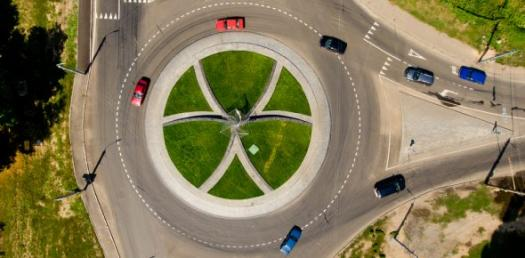# 2,3 / 2,4 Reading Quiz - Work Done, PE Ke, Power, Circular Motion

10 Questions | Attempts: 127
ShareSettings2,3 / 2,4 Reading Quiz - work done, PE KE, power, circular motion

• 1.
20N pushes a box 6m in 3 seconds. What is the work done by the force?
• A.

360J

• B.

120J

• C.

60J

• D.

18J

• 2.
What is the average power used in moving an object with 20N for a distance of 6m in 3seconds?
• A.

3.33W

• B.

2W

• C.

6.66W

• D.

40W

• 3.
What aspect of a force distance graph give you the work done?
• A.

• B.

The area

• C.

Half the area

• D.

The second derivative

• 4.
2Kg mass is thrown with a velocity of 5m/s.  What is its KE?
• A.

5J

• B.

10J

• C.

25J

• D.

50J

• 5.
A 2Kg mass is given 100J of KE.  How high will it reach if it is thrown vertically upwards?
• A.

2.5m

• B.

5m

• C.

25m

• D.

50m

• 6.
What is an elastic collision?
• A.

Where momentum is conserved

• B.

Where energy is conserved

• C.

Where kinetic energy is conserved

• D.

Where potential energy is conserved

• 7.
A speed boat is able to travel with a maximum velocity of 20m/s.  The power rating of the engine is 2000W.  What is the sum of the frictional forces on the boat?
• A.

40,000 N

• B.

200 N

• C.

100 N

• D.

50 N

• 8.
What is the acceleration of a 40Kg boy sitting on the edge of a merry-go-round.  The tangetial speed is 2m/s.  The diameter of the merry go round is 8m.  What is the acceleration he experiences.
• A.

0.5 m/s2

• B.

1m/s2

• C.

2m/s2

• D.

4m/s2

• 9.
When a bucket is being swung round in a circle in a verticle plane, what are the forces acting on the bucket when it is at the lowest point?
• A.

Weight and centripetal force,

• B.

Tension and centripetal force and weight

• C.

Weight and tension

• D.

Tension and centripetal force

• 10.
Which areas of this work done, KE, PE, power and circular motion do you have the most difficult with?  Can you formulate a question?
• 11.Back to top